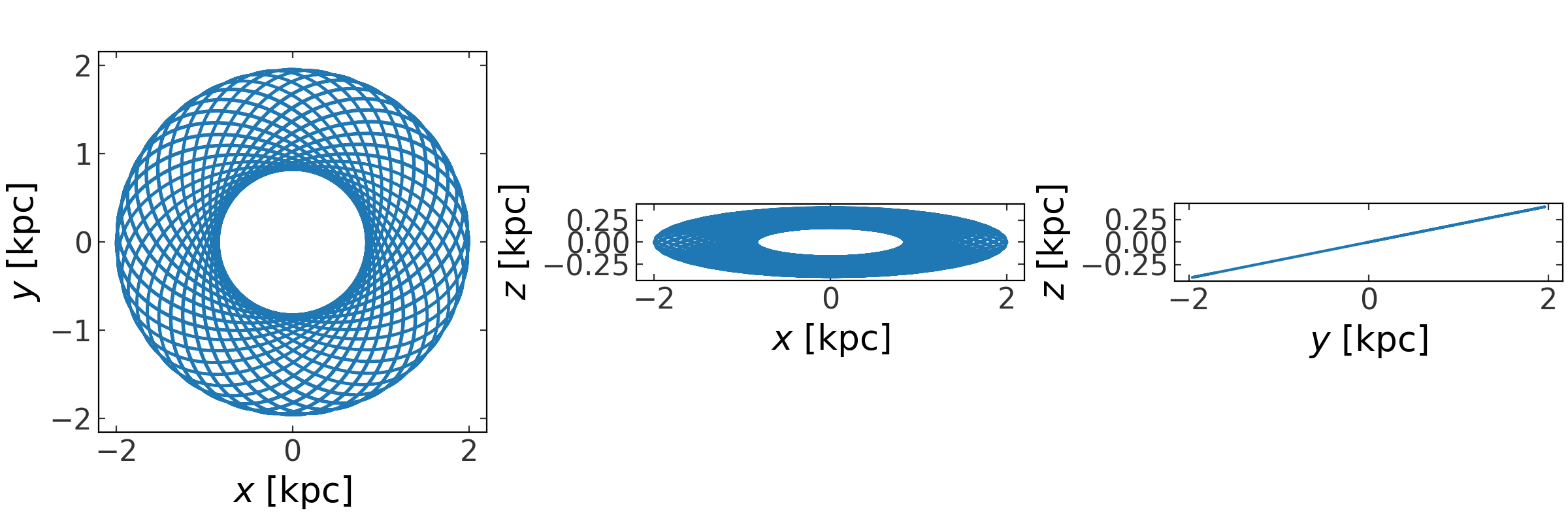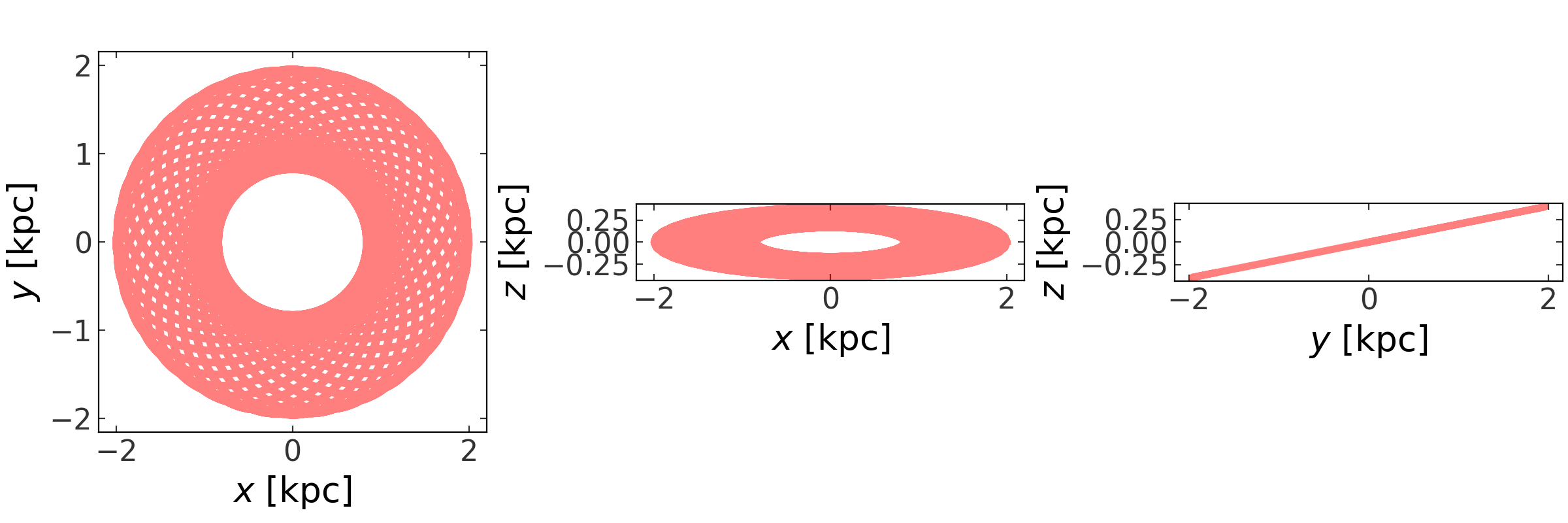# Orbit and phase-space position objects in more detail¶

For the examples below the following imports have already been executed:

```>>> import astropy.units as u
>>> import numpy as np
>>> import gala.potential as gp
>>> import gala.dynamics as gd
>>> from astropy.coordinates import (CylindricalRepresentation,
...                                  CylindricalDifferential)
>>> from gala.units import galactic
>>> np.random.seed(42)
```

We will also set the default Astropy Galactocentric frame parameters to the values adopted in Astropy v4.0:

```>>> import astropy.coordinates as coord
>>> _ = coord.galactocentric_frame_defaults.set('v4.0')
```

## Introduction¶

The `astropy.units` subpackage is excellent for working with numbers and associated units, but dynamical quantities often contain many quantities with mixed units. An example is a position in phase-space, which may contain some quantities with length units and some quantities with velocity or momentum units. The `PhaseSpacePosition` and `Orbit` classes are designed to work with these data structures and provide a consistent API for visualizing and computing further dynamical quantities. Click these shortcuts to jump to a section below, or start reading below:

## Phase-space Positions¶

The `PhaseSpacePosition` class provides an interface for representing full phase-space positions–coordinate positions and momenta (velocities). This class is useful as a container for initial conditions and for transforming phase-space positions to new coordinate representations or reference frames.

The easiest way to create a `PhaseSpacePosition` object is to pass in a pair of `Quantity` objects that represent the Cartesian position and velocity vectors:

```>>> gd.PhaseSpacePosition(pos=[4., 8., 15.] * u.kpc,
...                       vel=[-150., 50., 15.] * u.km/u.s)
<PhaseSpacePosition cartesian, dim=3, shape=()>
```

By default, passing in `Quantity`’s are interpreted as Cartesian coordinates and velocities. This works with arrays of positions and velocities as well:

```>>> x = np.arange(24).reshape(3, 8)
>>> v = np.arange(24).reshape(3, 8)
>>> w = gd.PhaseSpacePosition(pos=x * u.kpc,
...                           vel=v * u.km/u.s)
>>> w
<PhaseSpacePosition cartesian, dim=3, shape=(8,)>
```

This is interpreted as 8, 6-dimensional phase-space positions.

The class internally stores the positions and velocities as `BaseRepresentation` and `BaseDifferential` subclasses; in this case, `CartesianRepresentation` and `CartesianDifferential`:

```>>> w.pos
<CartesianRepresentation (x, y, z) in kpc
[(0.,  8., 16.), (1.,  9., 17.), (2., 10., 18.), (3., 11., 19.),
(4., 12., 20.), (5., 13., 21.), (6., 14., 22.), (7., 15., 23.)]>
>>> w.vel
<CartesianDifferential (d_x, d_y, d_z) in km / s
[(0.,  8., 16.), (1.,  9., 17.), (2., 10., 18.), (3., 11., 19.),
(4., 12., 20.), (5., 13., 21.), (6., 14., 22.), (7., 15., 23.)]>
```

All of the components of these classes are mapped to attributes of the phase-space position class for convenience, but with more user-friendly names. These mappings are defined in the class definition of `PhaseSpacePosition`. For example, to access the `x` component of the position and the `v_x` component of the velocity:

```>>> w.x
<Quantity [0.,1.,2.,3.,4.,5.,6.,7.] kpc>
>>> w.v_x
<Quantity [0.,1.,2.,3.,4.,5.,6.,7.] km / s>
```

The default representation is Cartesian, but the class can also be instantiated with representation objects instead of `Quantity`’s – this is useful for creating `PhaseSpacePosition` or `Orbit` instances from non-Cartesian representations of the position and velocity:

```>>> pos = CylindricalRepresentation(rho=np.linspace(1., 4, 4) * u.kpc,
...                                 phi=np.linspace(0, np.pi, 4) * u.rad,
...                                 z=np.linspace(-1, 1., 4) * u.kpc)
>>> vel = CylindricalDifferential(d_rho=np.linspace(100, 150, 4) * u.km/u.s,
...                               d_phi=np.linspace(-1, 1, 4) * u.rad/u.Myr,
...                               d_z=np.linspace(-15, 15., 4) * u.km/u.s)
>>> w = gd.PhaseSpacePosition(pos=pos, vel=vel)
>>> w
<PhaseSpacePosition cylindrical, dim=3, shape=(4,)>
>>> w.rho
<Quantity [1., 2., 3., 4.] kpc>
```

We can easily transform the full phase-space vector to new representations or coordinate frames. These transformations use the `astropy.coordinates` representations framework:

```>>> cart = w.represent_as('cartesian')
>>> cart.x
<Quantity [ 1. ,  1. , -1.5, -4. ] kpc>
>>> sph = w.represent_as('spherical')
>>> sph.distance
<Distance [1.41421356, 2.02758751, 3.01846171, 4.12310563] kpc>
```

There is also support for transforming the positions and velocities (assumed to be in a `Galactocentric` frame) to any of the other coordinate frames. For example, to transform to `Galactic` coordinates:

```>>> from astropy.coordinates import Galactic
>>> gal_c = w.to_coord_frame(Galactic)
>>> gal_c
<Galactic Coordinate: (l, b, distance) in (deg, deg, kpc)
[(4.40971301e-05, -6.23850462, 9.17891228),
(1.07501936e+01, -2.04017409, 9.29170644),
(2.14246214e+01,  2.65220588, 7.12026744),
(7.35169893e-05, 13.50991169, 4.23668468)]
(pm_l_cosb, pm_b, radial_velocity) in (mas / yr, mas / yr, km / s)
[( -28.11596908, -0.297625  ,    89.093095  ),
( -13.077309  ,  0.15891073,   511.60269726),
(  -7.04751509,  1.33976418, -1087.52574084),
(-206.97042166,  2.22471526,  -156.82064814)]>
```

We can easily plot projections of the phase-space positions using the `plot` method:

```>>> np.random.seed(42)
>>> x = np.random.uniform(-10, 10, size=(3,128))
>>> v = np.random.uniform(-200, 200, size=(3,128))
>>> w = gd.PhaseSpacePosition(pos=x * u.kpc,
...                           vel=v * u.km/u.s)
>>> fig = w.plot()
```This is a thin wrapper around the `plot_projections` function and any keyword arguments are passed through to that function:

```>>> fig = w.plot(components=['x', 'v_z'], color='r',
...              facecolor='none', marker='o', s=20, alpha=0.5)
```

## Orbits¶

The `Orbit` class inherits much of the functionality from `PhaseSpacePosition` (described above) and adds some additional features that are useful for time-series orbits.

An `Orbit` instance is initialized like the `PhaseSpacePosition`–with arrays of positions and velocities– but usually also requires specifying a time array as well. Also, the extra axes in these arrays hold special meaning for the `Orbit` class. The position and velocity arrays passed to `PhaseSpacePosition` can have arbitrary numbers of dimensions as long as the 0th axis specifies the dimensionality. For the `Orbit` class, the 0th axis remains the axis of dimensionality, but the 1st axis now is always assumed to be the time axis. For example, an input position with shape `(2,128)` to a `PhaseSpacePosition` represents 128 independent 2D positions, but to a `Orbit` it represents a single orbit’s positions at 128 times:

```>>> t = np.linspace(0, 100, 128) * u.Myr
>>> Om = 1E-1 * u.rad / u.Myr
>>> pos = np.vstack((5*np.cos(Om*t), np.sin(Om*t))).value * u.kpc
>>> vel = np.vstack((-5*np.sin(Om*t), np.cos(Om*t))).value * u.kpc/u.Myr
>>> orbit = gd.Orbit(pos=pos, vel=vel)
>>> orbit
<Orbit ndcartesian, dim=2, shape=(128,)>
```

To create a single object that contains multiple orbits, the input position object should have 3 axes. The last axis (`axis=2`) specifies the number of orbits. So, an input position with shape `(2,128,16)` would represent 16, 2D orbits, each with the same 128 times:

```>>> t = np.linspace(0, 100, 128) * u.Myr
>>> Om = np.random.uniform(size=16) * u.rad / u.Myr
>>> angle = Om[None] * t[:, None]
>>> pos = np.stack((5*np.cos(angle), np.sin(angle))).value * u.kpc
>>> vel = np.stack((-5*np.sin(angle), np.cos(angle))).value * u.kpc/u.Myr
>>> orbit = gd.Orbit(pos=pos, vel=vel)
>>> orbit
<Orbit ndcartesian, dim=2, shape=(128, 16)>
```

To make full use of the orbit functionality, you must also pass in an array with the time values and an instance of a `PotentialBase` subclass that represents the potential that the orbit was integrated in:

```>>> pot = gp.PlummerPotential(m=1E10, b=1., units=galactic)
>>> orbit = gd.Orbit(pos=pos*u.kpc, vel=vel*u.km/u.s,
...                  t=t*u.Myr, potential=pot)
```

(note, in this case `pos` and `vel` were not generated from integrating an orbit in the potential `pot`!). However, most of the time you won’t need to create `Orbit` objects from scratch! They are returned from any of the numerical integration routines provided in `gala`. For example, they are returned by the `integrate_orbit` method of potential objects and will automatically contain the `time` array and `potential` object. For example:

```>>> pot = gp.PlummerPotential(m=1E10 * u.Msun, b=1. * u.kpc, units=galactic)
>>> w0 = gd.PhaseSpacePosition(pos=[10.,0,0] * u.kpc,
...                            vel=[0.,75,0] * u.km/u.s)
>>> orbit = gp.Hamiltonian(pot).integrate_orbit(w0, dt=1., n_steps=5000)
>>> orbit
<Orbit cartesian, dim=3, shape=(5001,)>
>>> orbit.t
<Quantity [0.000e+00, 1.000e+00, 2.000e+00, ..., 4.998e+03, 4.999e+03,
5.000e+03] Myr>
>>> orbit.potential
```

Just like for `PhaseSpacePosition`, we can quickly visualize an orbit using the `plot` method:

```>>> fig = orbit.plot()
```Again, this is a thin wrapper around the `plot_projections` function and any keyword arguments are passed through to that function:

```>>> fig = orbit.plot(linewidth=4., alpha=0.5, color='r')
```We can also quickly compute quantities like the angular momentum, and estimates for the pericenter, apocenter, eccentricity of the orbit. Estimates for the latter few get better with smaller timesteps:

```>>> orbit = gp.Hamiltonian(pot).integrate_orbit(w0, dt=0.1, n_steps=100000)
>>> np.mean(orbit.angular_momentum(), axis=1)
<Quantity [0.        ,0.        ,0.76703412] kpc2 / Myr>
>>> orbit.eccentricity()
<Quantity 0.31951765618193967>
>>> orbit.pericenter()
<Quantity 10.00000005952518 kpc>
>>> orbit.apocenter()
<Quantity 19.390916871970223 kpc>
```

Internally, both of the above classes rely on the Astropy representation transformation framework (i.e. the subclasses of `BaseRepresentation` and `BaseDifferential`). However, at present these classes only support 3D positions and differentials (velocities). The `PhaseSpacePosition` and `Orbit` classes both support arbitrary numbers of dimensions and, when relevant, rely on custom subclasses of the representation classes to handle such cases. See the N-dimensional representation classes page for more information about these classes.